# Engineering Mechanics - Force System Resultants

### Exercise :: Force System Resultants - General Questions

• Force System Resultants - General Questions
16.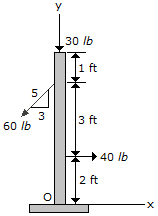Replace the loading system acting on the post by an equivalent force and couple system at point O.

 A. F = (-8i-66j) lb, M = 220 lb-ft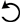B. F = (-8i-66j) lb, M = 160 lb-ftC. F = (4i78j) lb, M = 220 lb-ftD. F = (4i78j) lb, M = 100 lb-ftExplanation:

No answer description available for this question. Let us discuss.

17.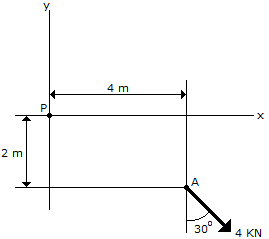Determine the magnitude and direction of the moment of the force of the movement if the force at A about point P.

 A. Mp = 17.86 kNmB. Mp = 1.072 kNmC. Mp = 9.86 kNm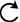D. Mp = 14.93 kNmExplanation:

No answer description available for this question. Let us discuss.

18.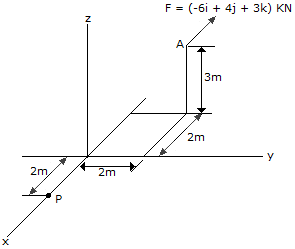Determine the moment of the force at A about point P. Use a vector analysis and express the result in Cartesian vector form.

 A. MP = (-6i+6j-4k) N-m B. MP = (24i+8j+9k) N-m C. MP = (-6i-6j-4k) N-m D. MP = (24i-8j +9k) N-m

Explanation:

No answer description available for this question. Let us discuss.

19.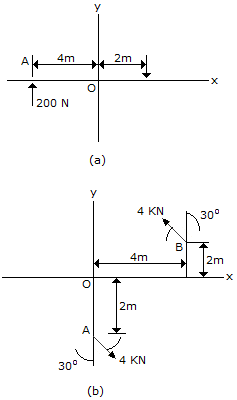Determine the magnitude and direction of the couple shown.

 A. M = 1200 N-m CW B. M = 400 N-m CCW C. M = 400 N-m CW D. M = 1200 N-m CCW

Explanation:

No answer description available for this question. Let us discuss.

20.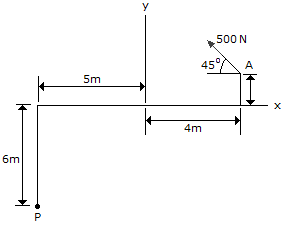Determine the magnitude and direction of the moment of the force at A about point P.

 A. Mp = 0.707 kN-mB. Mp = 5.66 kN-mC. Mp = 5.70 kN-mD. Mp = 4.50 kN-m